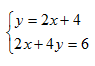Substitution

When graphing is not a viable option, systems of equations can be solved symbolically. One method for a symbolic solution is substitution. When solving linear systems using substitution, one variable must be replaced with an equivalent expression that includes the other variable.

Example 1 Solve the following system by substitution.Step 1. Isolate one variable in one equation.

Since y is isolated in the first equation, use that variable and that equation.

Step 2. Substitute the isolated variable's equivalent expression into the other equation.

Since y is equal to 2x + 4, substitute the "y" in 2x + 4y = 6, with the equivalent expression, 2x +4.

2x + 4(2x + 4) = 6

Step 3. Solve the remaining equation.

2x + 8x + 16 = 6

10x +16 = 6

10x = -10

x = -1

Step 4. Substitute the value of the variable found in step 3, into either equation to find the other variable.

y = 2(-1) + 4

y = -2 + 4

y = 2

Therefore the solution point is (-1, 2).

Listen